# Colleges with the highest SAT scores in South Dakota

Top 9 colleges in South Dakota with the highest SAT scores
Looking for the colleges with the highest SAT scores in South Dakota? Well you're in luck! We've compiled a national college database and have created a list of the top 9 universities with the highest SAT scores in South Dakota. These are the schools whose applicants had the highest average SAT scores in South Dakota. And, since these tests are meant to determine academic prowess, they are arguably the schools with the most academically proficient students. You could even say these are the best colleges in South Dakota. We also include each college's ACT scores and acceptance rate so that you can see where you would have the easiest or hardest time getting in. Read on to find out more.

## South Dakota School of Mines and Technology SAT scores

The average SAT score for South Dakota School of Mines and Technology is 1220.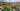The average SAT score of 1220 breaks down into:

• SAT math: 650

The average ACT score for South Dakota School of Mines and Technology is 27 and their acceptance rate is 84.5%.

## University of South Dakota SAT scores

The average SAT score for University of South Dakota is 1151.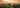The average SAT score of 1151 breaks down into:

• SAT math: 580

The average ACT score for University of South Dakota is 23 and their acceptance rate is 85.8%.

## Mount Marty College SAT scores

The average SAT score for Mount Marty College is 1110.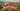The average SAT score of 1110 breaks down into:

• SAT math: 555

The average ACT score for Mount Marty College is 21 and their acceptance rate is 69.2%.

## South Dakota State University SAT scores

The average SAT score for South Dakota State University is 1110.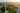The average SAT score of 1110 breaks down into:

• SAT math: 570

The average ACT score for South Dakota State University is 23 and their acceptance rate is 90.8%.

## University of Sioux Falls SAT scores

The average SAT score for University of Sioux Falls is 1108.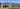The average SAT score of 1108 breaks down into:

• SAT math: 550

The average ACT score for University of Sioux Falls is 23 and their acceptance rate is 91.3%.

## Northern State University SAT scores

The average SAT score for Northern State University is 1098.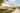The average SAT score of 1098 breaks down into:

• SAT math: 570

The average ACT score for Northern State University is 21 and their acceptance rate is 88.4%.

## Dakota State University SAT scores

The average SAT score for Dakota State University is 1090.The average SAT score of 1090 breaks down into:

• SAT math: 550

The average ACT score for Dakota State University is 23 and their acceptance rate is 83.2%.

## Black Hills State University SAT scores

The average SAT score for Black Hills State University is 1080.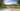The average SAT score of 1080 breaks down into:

• SAT math: 540

The average ACT score for Black Hills State University is 21 and their acceptance rate is 87%.

## Dakota Wesleyan University SAT scores

The average SAT score for Dakota Wesleyan University is 1080.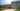The average SAT score of 1080 breaks down into:

• SAT math: 540